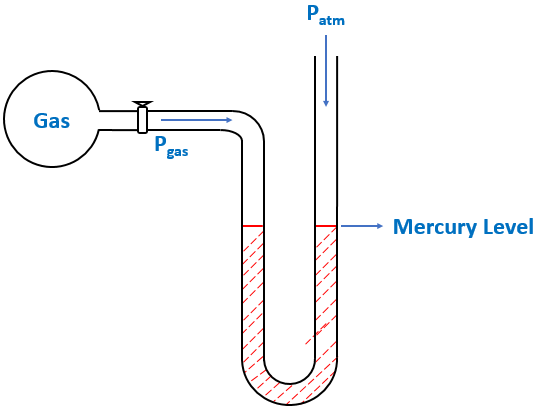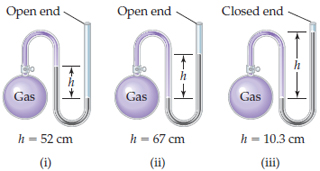# Problem: If the atmospheric pressure is 0.980 atm , what is the pressure of the enclosed gas in each of the three cases depicted in the drawing ?What is the pressure of the enclosed gas in (ii)? Assume that the gray liquid is mercury.

###### FREE Expert Solution

We’re being asked to determine the pressure of a gas using an open-end manometer.

A manometer allows you to determine the pressure of a gas (Pgas) sample by using the atmospheric pressure (Patm) and the difference in the height of mercury (h).

Different conditions for an open-end manometer are illustrated below:

When Pgas is pushing the same force as Patm$\overline{){{\mathbf{P}}}_{\mathbf{g}\mathbf{a}\mathbf{s}}{\mathbf{=}}{{\mathbf{P}}}_{\mathbf{a}\mathbf{t}\mathbf{m}}}$

When Pgas is pushing harder than Patm

97% (211 ratings)###### Problem Details

If the atmospheric pressure is 0.980 atm , what is the pressure of the enclosed gas in each of the three cases depicted in the drawing?

What is the pressure of the enclosed gas in (ii)? Assume that the gray liquid is mercury.